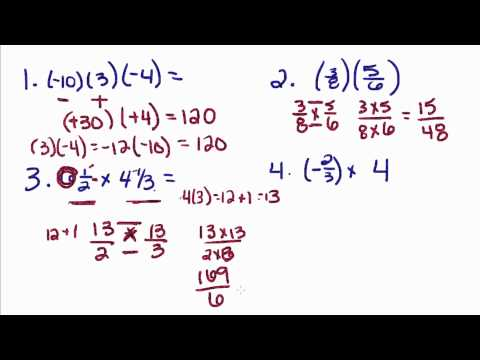# thenewboston► Play all

# Algebra Tutorials Playlist

• thenewboston
• 32 videos
• 72,310 views
• Last updated on Jun 27, 2014
thenewboston Official Algebra Tutorial Playlist!

Loading...

•  Algebra Tutorial - 1 - Multiplying and Dividing Real Numbers 9:58 Algebra Tutorial - 2 - Order of Operations 7:24 Algebra Tutorial - 3 - Opposite and Absolute Values 4:33 Algebra Tutorial - 4 - Combining Like Terms 5:05 Algebra Tutorial - 5 - Solving Equations with Addition and Subtraction 6:14 Algebra Tutorial - 6 - Solving Equations with Multiplication and Division 4:23 Algebra Tutorial - 7 - Solving Combined Equations 4:28 Algebra Tutorial - 8 - Solving Equations with Variables on Both Sides 5:41 Algebra Tutorial - 9 - Solving Word Problems with Equations 7:37 Algebra Tutorial - 10 - Solving and Graphing Basic Inequalities 9:11 Algebra Tutorial - 11 - Solving and Graphing Compound Inequalities 7:34 Algebra Tutorial - 12 - Exponents 6:54 Algebra Tutorial - 13 - Adding and Subtracting Polynomials 6:50 Algebra Tutorial - 14 - Multiplying a Polynomial by a Monomial 7:41 Algebra Tutorial - 15 - Multiplying Binomials 4:37 Algebra Tutorial - 16 - Multiplying Polynomials 7:03 Algebra Tutorial - 17 - Special Products of Binomials 3:17 Algebra Tutorial - 18 - Squaring Binomials 3:40 Algebra Tutorial - 19 - Polynomial Equations 6:07 Algebra Tutorial - 20 - Solving Geometry Problems with Polynomials 7:16 Algebra Tutorial - 21 - Solving Word Problems with Polynomials 8:13 Algebra Tutorial - 22 - Greatest Common Factor 9:41 Algebra Tutorial - 23 - Factoring the Difference of Squares 6:28 Algebra Tutorial - 24 - Factoring Perfect Square Trinomials 4:41 Algebra Tutorial - 25 - Factoring Trinomials 6:05 Algebra Tutorial - 26 - Solving Equations- Factoring 6:59 Algebra Tutorial - 27 - Factoring- Word Problems 6:54 Algebra Tutorial - 28 - Factoring- Geometry Problems 6:16 Algebra Tutorial - 29 - Dividing Monomials 3:58 Algebra Tutorial - 30 - Dividing a Polynomial by a Monomial 4:04 Algebra Tutorial - 31 - Simplifying Fractions 4:45 Algebra Tutorial - 32 - Multiplying Fractions 4:51
to add this to Watch Later

### Add to

Loading playlists...